Processing ......FreeComputerBooks.com Links to Free Computer, Mathematics, Technical Books all over the World

Graph Theory
Related Book Categories:
•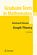Graph Theory (Reinhard Diestel)

This book covers the core material of the subject with concise yet reliably complete proofs, while offering glimpses of more advanced methods in each field by one or two deeper results, again with proofs given in full detail.

•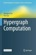Hypergraph Computation (Qionghai Dai, et al.)

Comprehensive and systematic overview for Hypergraph computation. Rich blend of basic knowledge, theoretical analysis, algorithm introduction, and key applications. Describes hypergraph computation applications in computer vision, medical applications, etc.

•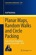Planar Maps, Random Walks and Circle Packing (Asaf Nachmias)

This open access book focuses on the interplay between random walks on planar maps and Koebe’s circle packing theorem. Entirely self-contained. Many classical proofs have been simplified and streamlined. Contains numerous useful exercises.

•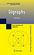Digraphs: Theory, Algorithms and Applications (J. Bang-Jensen)

This book is an essential, comprehensive reference of Digraphs covering the theoretical aspects of the subject, focus on applications which include quantum mechanics, bioinformatics, embedded computing, and the travelling salesman problem.

•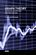Graph Theory - Advanced Algorithms and Applications

Not only will the methods and explanations help you to understand more about graph theory, but you will find it joyful to discover ways that you can apply graph theory in your applications or scientific research.

•Graph Theory and Complex Networks (Maarten van Steen)

This book aims to explain the basics of graph theory that are needed at an introductory level for students in computer or information sciences. It also aims to provide an introduction to the modern field of network science.

•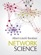Network Science (Albert-László Barabási)

Illustrated throughout in full colour, this pioneering textbook, spanning a wide range of topics from physics to computer science, engineering, economics and the social sciences, introduces network science to an interdisciplinary audience.

•Probability on Trees and Networks (Russell Lyons, et al.)

This book is concerned with certain aspects of discrete probability on infinite graphs that are currently in vigorous development. Of course, finite graphs are analyzed as well, but usually with the aim of understanding infinite graphs and networks.

•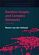Random Graphs and Complex Networks (Remco van der Hofstad)

This rigorous introduction to network science presents Random Graphs as models for real-world networks. Such networks have distinctive empirical properties and a wealth of new models have emerged to capture them.

•Graph Algorithms: Practical Examples in Apache Spark and Neo4j

This book is a practical guide to getting started with graph algorithms for developers and data scientists who have experience using Apache Spark or Neo4j. You'll walk through hands-on examples that show you how to use graph algorithms in Apache Spark/Neo4j.

•A Survey of Statistical Network Models (Anna Goldenberg, et al.)

This book aims to provide the reader with an entry point to the voluminous literature on statistical network modeling. It guides the reader through the development of key stochastic network models, touches upon a number of examples and commonalities.

•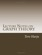Lecture Notes on Graph Theory (Tero Harju)

These are introductory lecture notes on graph theory. It offers undergraduates a remarkably student-friendly introduction to graph theory and takes an engaging approach that emphasizes graph theory's history.

•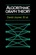Algorithmic Graph Theory (David Joyner, et al)

This is an introductory book on algorithmic graph theory. Theory and algorithms are illustrated using the Sage open source mathematics software. It's especially suitable for computer scientists and mathematicians interested in computational complexity.

•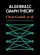Explorations in Algebraic Graph Theory (Chris Godsil, et al.)

This book aims to express properties of graphs in algebraic terms, then to deduce theorems about them. It tackles the applications of linear algebra and matrix theory to the study of graphs; algebraic constructions such as adjacency matrix, using the Sage.

•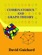An Introduction to Combinatorics and Graph Theory

This book walks the reader through the classic parts of Combinatorics and graph theory, while also discussing some recent progress in the area: on the one hand, providing material that will help students learn the basic techniques.

•Graph Databases: New Opportunities for Connected Data

This book provides a practical foundation for those who want to apply Graph Database to real-world business solutions. You'll learn why graph database are useful, where they're applicable, and how to design and implement solutions that use them.

•Advances in Graph Algorithms (Ton Kloks, et al.)

This is a book about some currently popular topics such as exponential algorithms, fixed-parameter algorithms and algorithms using decomposition trees of graphs which is one of focuses of the book - occupied a whole chapter.

•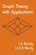Graph Theory with Applications (J.A. Bondy, et al.)

The primary aim of this book is to present a coherent introduction to graph theory, suitable as a textbook for advanced undergraduate and beginning graduate students in mathematics and computer science.

•Graph Theory Lessons (Christopher P. Mawata)

This text covers the important elementary topics of graph theory and its apps. It utilizes a java software as an investigative tool which can draw, edit and manipulate simple graphs, examine properties of the graphs, and demonstrate them using computer animation.

•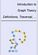Introduction to Graph Theory: Definitions, Traversal, Analysis, etc.

Requiring only high school algebra as math background, it leads the reader from simple graphs through planar graphs, Euler's formula, Platonic graphs, coloring, the genus of a graph, Euler walks, Hamilton walks, and a discussion of The Seven Bridges of Konigsberg.

•New Frontiers in Graph Theory (Yagang Zhang)

The purpose of this book is not only to present the latest state and development tendencies of graph theory, but to bring the reader far enough along the way to enable him to embark on the research problems of his own.

•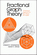Fractional Graph Theory: A Rational Approach (E. Scheinerman)

In this book the authors explore generalizations of core graph theory notions by allowing real values to substitute where normally only integers would be permitted. The aim is to prove "fractional analogues" of the theorems of traditional graph theory.

•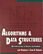Algorithms and Data Structures: Apps to Graphics and Geometry

An introductory coverage of algorithms and data structures with application to graphics and geometry aims to show a sample of the intellectual demands required by a computer science curriculum, exercises, many with solutions, are included throughout the book.

•Lists, Decisions and Graphs - With an Introduction to Probability

In this book, four basic areas of discrete mathematics are presented: Counting and Listing (Unit CL), Functions (Unit Fn), Decision Trees and Recursion (Unit DT), and Basic Concepts in Graph Theory (Unit GT).

•Introductory Map Theory (Yanpei Liu)

As an introductory book, this book contains the elementary materials in map theory, including embeddings of a graph, abstract maps, duality, orientable and non-orientable maps, isomorphisms of maps and the enumeration of rooted or unrooted maps, etc.

•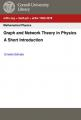Graph and Network Theory in Physics: A Short Introduction

It consists of some of the main areas of research in graph and network theory applied to physics, includes graphs in condensed matter theory, such as the tight-binding and the Hubbard model and statistical physics by means of the analysis of the Potts model ...

•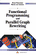Functional Programming and Parallel Graph Rewriting

This is an introduction to the techniques of functional programming, focusing on an alternative computational model - Graph Rewriting Systems. The book demonstrates and discusses the potential power of concurrent functional programming and the implementation on loosely coupled parallel architectures.

•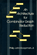Architecture for Combinator Graph Reduction (Philip J. Koopman)

The results of cache-simulation experiments with an abstract machine for reducing combinator graphs are presented, exhibits reduction rates that, for similar kinds of combinator graphs on similar kinds of hardware, compare favorably with previously reported techniques.

•Knowledge Graphs and Big Data Processing (Valentina Janev, et al)

Each chapter in this book addresses some pertinent aspect of the data processing chain, with a specific focus on understanding Enterprise Knowledge Graphs, Semantic Big Data Architectures, and Smart Data Analytics solutions.

•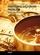Traveling Salesman Problem, Theory and Applications

This book is a collection of current research in the application of evolutionary algorithms and other optimal algorithms to solving the TSP problem, including Artificial Immune Systems, Genetic Algorithms, Neural Networks and Differential Evolution Algorithm.

•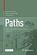Paths: Why is Life Filled with so many Detours?

This book explores the amazing similarity between paths taken by people and many other things in life, and its impact on the way we live, teach and learn. Offering insights into the new scientific field of paths as part of the science of networks, etc.

•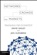Networks, Crowds, and Markets: a Highly Connected World

It looks at economics, sociology, computing and information science, and applied mathematics to understand networks and behavior, and addresses fundamental questions about how the social, economic, and technological worlds are connected.

•O'Reilly® Graph Databases: New Opportunities for Connected Data

This book provides a practical foundation for those who want to apply Graph Database to real-world business solutions. You'll learn why graph database are useful, where they're applicable, and how to design and implement solutions that use them.

Book Categories
 :All CategoriesTop Free BooksRecent BooksMiscellaneous BooksComputer EngineeringComputer LanguagesComputer ScienceData Science/DatabasesJava and Java EE (J2EE)Linux and UnixMathematicsMicrosoft and .NETMobile ComputingNetworking and CommunicationsSoftware EngineeringSpecial TopicsWeb Programming
Other Categories